Anzeige

# STEM_GC11PC-If-31.pptx

24. Nov 2022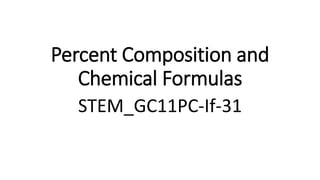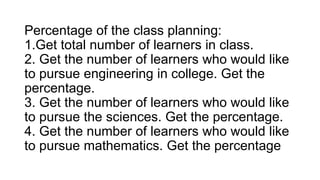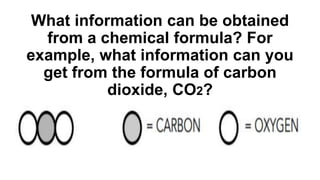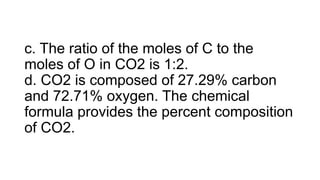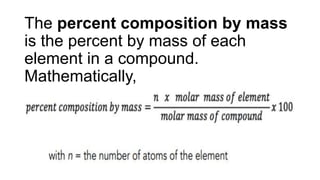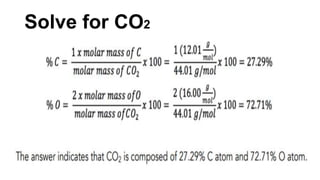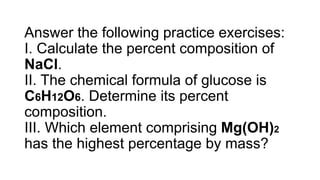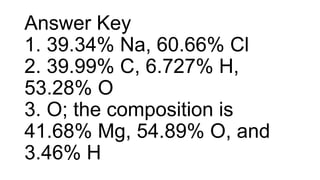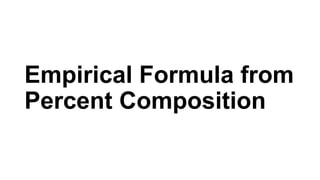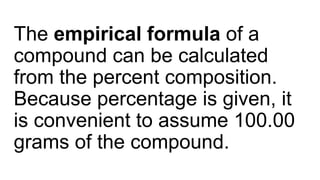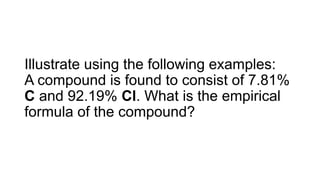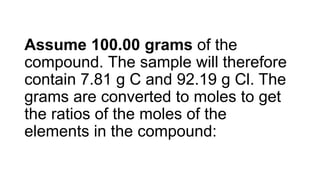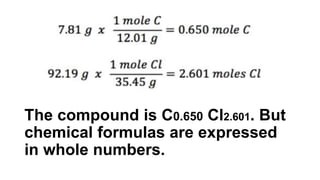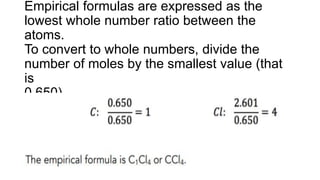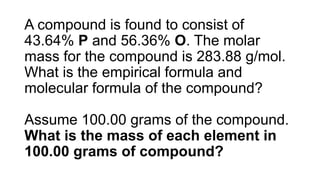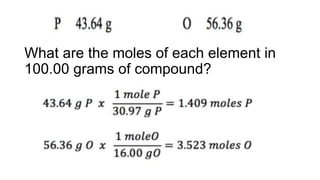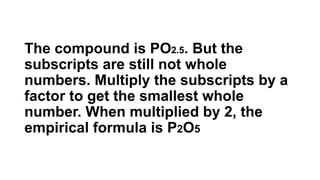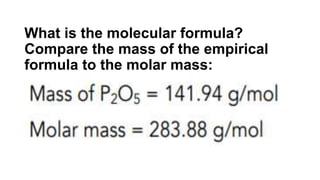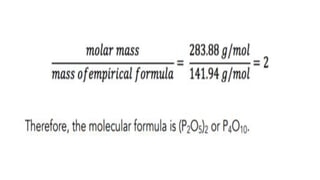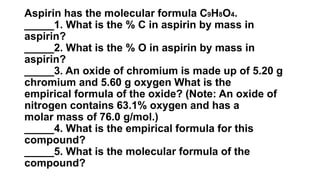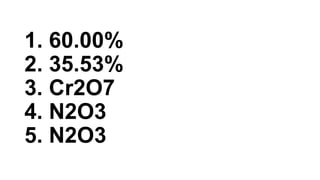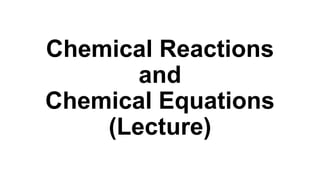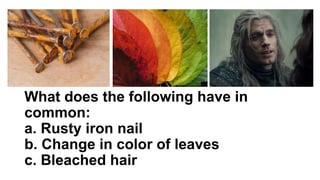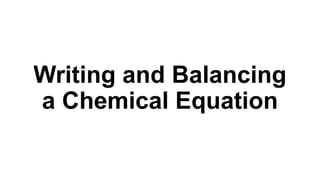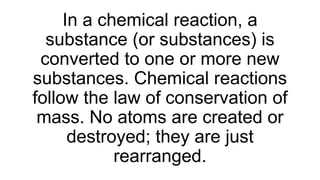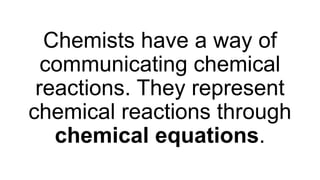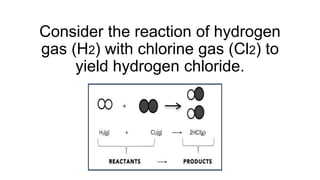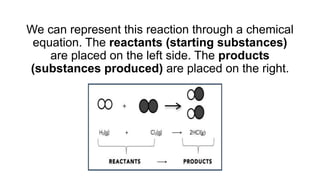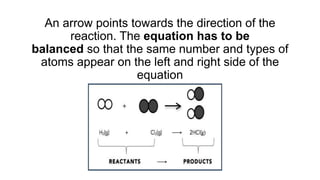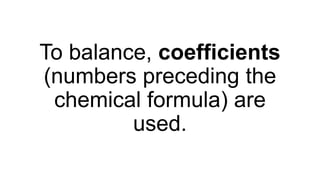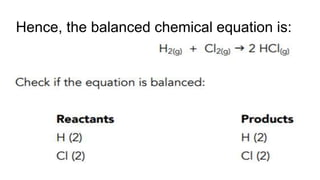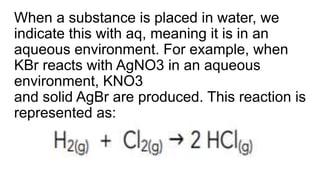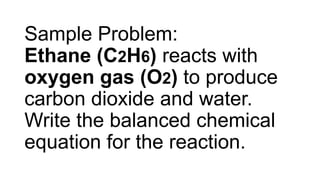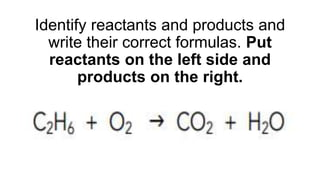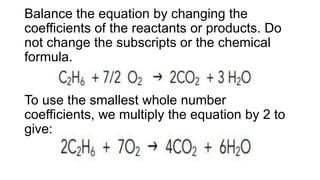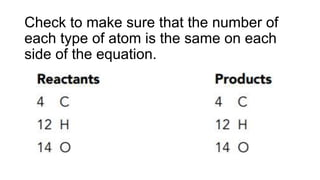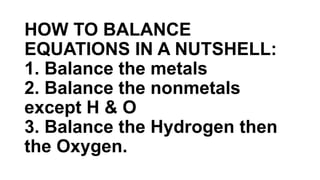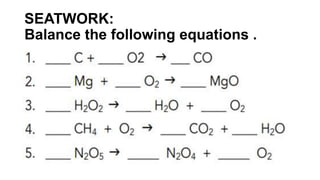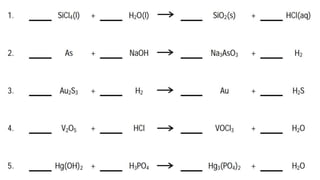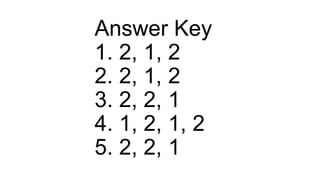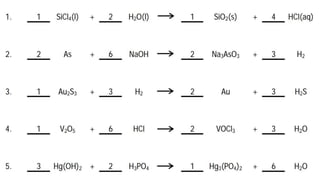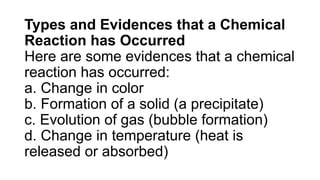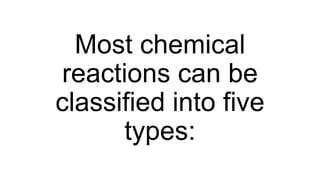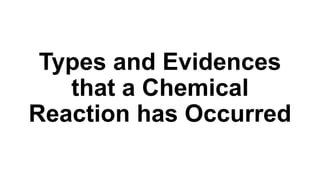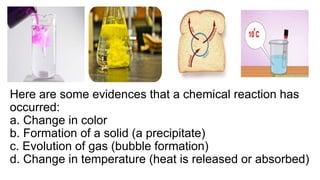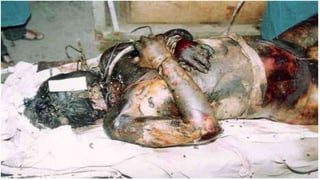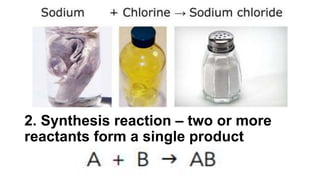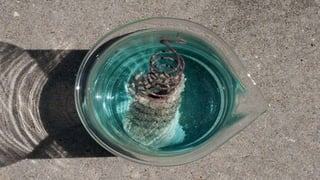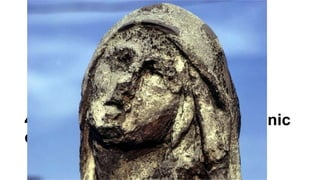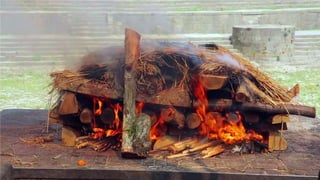1 von 56
Anzeige

### STEM_GC11PC-If-31.pptx

1. Percent Composition and Chemical Formulas STEM_GC11PC-If-31
2. Percentage of the class planning: 1.Get total number of learners in class. 2. Get the number of learners who would like to pursue engineering in college. Get the percentage. 3. Get the number of learners who would like to pursue the sciences. Get the percentage. 4. Get the number of learners who would like to pursue mathematics. Get the percentage
3. What information can be obtained from a chemical formula? For example, what information can you get from the formula of carbon dioxide, CO2?
4. a. The compound is made up of two elements, namely carbon and oxygen. b. One molecule of CO2 is made up of one atom of carbon and two atoms of oxygen. c. One mole of CO2 molecules will have one mole of C atoms and two moles of O atoms.
5. c. The ratio of the moles of C to the moles of O in CO2 is 1:2. d. CO2 is composed of 27.29% carbon and 72.71% oxygen. The chemical formula provides the percent composition of CO2.
6. The percent composition by mass is the percent by mass of each element in a compound. Mathematically,
7. Solve for CO2
8. Answer the following practice exercises: I. Calculate the percent composition of NaCl. II. The chemical formula of glucose is C6H12O6. Determine its percent composition. III. Which element comprising Mg(OH)2 has the highest percentage by mass?
9. Answer Key 1. 39.34% Na, 60.66% Cl 2. 39.99% C, 6.727% H, 53.28% O 3. O; the composition is 41.68% Mg, 54.89% O, and 3.46% H
10. Empirical Formula from Percent Composition
11. The empirical formula of a compound can be calculated from the percent composition. Because percentage is given, it is convenient to assume 100.00 grams of the compound.
12. Illustrate using the following examples: A compound is found to consist of 7.81% C and 92.19% Cl. What is the empirical formula of the compound?
13. Assume 100.00 grams of the compound. The sample will therefore contain 7.81 g C and 92.19 g Cl. The grams are converted to moles to get the ratios of the moles of the elements in the compound:
14. The compound is C0.650 Cl2.601. But chemical formulas are expressed in whole numbers.
15. Empirical formulas are expressed as the lowest whole number ratio between the atoms. To convert to whole numbers, divide the number of moles by the smallest value (that is 0.650).
16. A compound is found to consist of 43.64% P and 56.36% O. The molar mass for the compound is 283.88 g/mol. What is the empirical formula and molecular formula of the compound? Assume 100.00 grams of the compound. What is the mass of each element in 100.00 grams of compound?
17. What are the moles of each element in 100.00 grams of compound?
18. The compound is PO2.5. But the subscripts are still not whole numbers. Multiply the subscripts by a factor to get the smallest whole number. When multiplied by 2, the empirical formula is P2O5
19. What is the molecular formula? Compare the mass of the empirical formula to the molar mass:
20. Aspirin has the molecular formula C9H8O4. _____1. What is the % C in aspirin by mass in aspirin? _____2. What is the % O in aspirin by mass in aspirin? _____3. An oxide of chromium is made up of 5.20 g chromium and 5.60 g oxygen What is the empirical formula of the oxide? (Note: An oxide of nitrogen contains 63.1% oxygen and has a molar mass of 76.0 g/mol.) _____4. What is the empirical formula for this compound? _____5. What is the molecular formula of the compound?
21. 1. 60.00% 2. 35.53% 3. Cr2O7 4. N2O3 5. N2O3
22. Chemical Reactions and Chemical Equations (Lecture)
23. What does the following have in common: a. Rusty iron nail b. Change in color of leaves c. Bleached hair
24. Writing and Balancing a Chemical Equation
25. In a chemical reaction, a substance (or substances) is converted to one or more new substances. Chemical reactions follow the law of conservation of mass. No atoms are created or destroyed; they are just rearranged.
26. Chemists have a way of communicating chemical reactions. They represent chemical reactions through chemical equations.
27. Consider the reaction of hydrogen gas (H2) with chlorine gas (Cl2) to yield hydrogen chloride.
28. We can represent this reaction through a chemical equation. The reactants (starting substances) are placed on the left side. The products (substances produced) are placed on the right.
29. An arrow points towards the direction of the reaction. The equation has to be balanced so that the same number and types of atoms appear on the left and right side of the equation
30. To balance, coefficients (numbers preceding the chemical formula) are used.
31. Hence, the balanced chemical equation is:
32. When a substance is placed in water, we indicate this with aq, meaning it is in an aqueous environment. For example, when KBr reacts with AgNO3 in an aqueous environment, KNO3 and solid AgBr are produced. This reaction is represented as:
33. Sample Problem: Ethane (C2H6) reacts with oxygen gas (O2) to produce carbon dioxide and water. Write the balanced chemical equation for the reaction.
34. Identify reactants and products and write their correct formulas. Put reactants on the left side and products on the right.
35. Balance the equation by changing the coefficients of the reactants or products. Do not change the subscripts or the chemical formula. To use the smallest whole number coefficients, we multiply the equation by 2 to give:
36. Check to make sure that the number of each type of atom is the same on each side of the equation.
37. HOW TO BALANCE EQUATIONS IN A NUTSHELL: 1. Balance the metals 2. Balance the nonmetals except H & O 3. Balance the Hydrogen then the Oxygen.
38. SEATWORK: Balance the following equations .
39. Answer Key 1. 2, 1, 2 2. 2, 1, 2 3. 2, 2, 1 4. 1, 2, 1, 2 5. 2, 2, 1
40. Types and Evidences that a Chemical Reaction has Occurred Here are some evidences that a chemical reaction has occurred: a. Change in color b. Formation of a solid (a precipitate) c. Evolution of gas (bubble formation) d. Change in temperature (heat is released or absorbed)
41. Most chemical reactions can be classified into five types:
42. Types and Evidences that a Chemical Reaction has Occurred
43. Here are some evidences that a chemical reaction has occurred: a. Change in color b. Formation of a solid (a precipitate) c. Evolution of gas (bubble formation) d. Change in temperature (heat is released or absorbed)
44. What does the following have in common: a. Rusty iron nail b. Change in color of leaves c. Bleached hair
45. Most chemical reactions can be classified into five types: 1. Decomposition reaction – a reactant breaks down into two or more products
46. 2. Synthesis reaction – two or more reactants form a single product
47. 3. Single displacement reaction – one element replaces another in a compound
48. 4. Double displacement – two ionic compounds exchange ions
49. 5. Combustion reaction – a hydrocarbon (a compound containing carbon and hydrogen) reacts with oxygen to form carbon dioxide and water.

### Hinweis der Redaktion

1. Copper displaces dissolved silver from a solution. When a copper wire is dipped in a silver nitrate solution, solid silver precipitates out.
2. CaCO3 + 2HNO3 -> Ca(NO3)2 + H2O + CO2
Anzeige